# Quaternion

A hypercomplex number, geometrically realizable in four-dimensional space. The system of quaternions was put forward in 1843 by W.R. Hamilton (1805–1865). Quaternions were historically the first example of a hypercomplex system, arising from attempts to find a generalization of complex numbers. Complex numbers are depicted geometrically by points in the plane and operations on them correspond to the simplest geometric transformations of the plane. It is not possible to "organize" a number system similar to the field of real or complex numbers from the points of a space of three or more dimensions. However, if one drops the requirement of commutativity of multiplication, then it is possible to construct a number system from the points of-dimensional space. (In 3, 5 or higher-dimensional space it is not even possible to do this.)

The quaternions form a-dimensional algebra over the field of real numbers with basis( "basic units" ) and the following multiplication table of the "basic units" :

<tbody> </tbody>
 1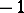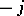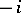Every quaternion can be written in the formor (since 1 plays the role of ordinary identity and in writing a quaternion it can be omitted) in the form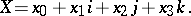One distinguishes the scalar partof the quaternion and its vector partso that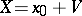. If, then the quaternionis called a vector and can be identified with an ordinary-dimensional vector, since multiplication in the algebra of quaternions of two such vectors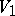and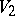is related to the scalar and vector products(cf. Inner product) and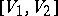(cf. Vector product) of the vectorsandin-dimensional space by the formulaThis shows the close relationship between quaternions and vector calculus. Historically, the latter arose from the theory of quaternions.

Corresponding to each quaternionis the conjugate quaternion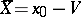, and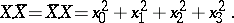This real number is called the norm of the quaternionand is denoted by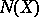. This norm satisfies the relation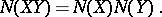Any rotation of-dimensional space about the origin can be defined by means of a quaternionwith norm 1. The rotation corresponding totakes the vector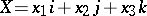to the vector.

The algebra of quaternions is the unique associative non-commutative finite-dimensional normed algebra over the field of real numbers with an identity. The algebra of quaternions is a skew-field, that is, division is defined in it, and the quaternion inverse to a quaternionis. The skew-field of quaternions is the unique finite-dimensional real associative non-commutative algebra without divisors of zero (see also Frobenius theorem; Cayley–Dickson algebra).

How to Cite This Entry:
Quaternion. Encyclopedia of Mathematics. URL: http://encyclopediaofmath.org/index.php?title=Quaternion&oldid=35148
This article was adapted from an original article by N.N. Vil'yams (originator), which appeared in Encyclopedia of Mathematics - ISBN 1402006098. See original article